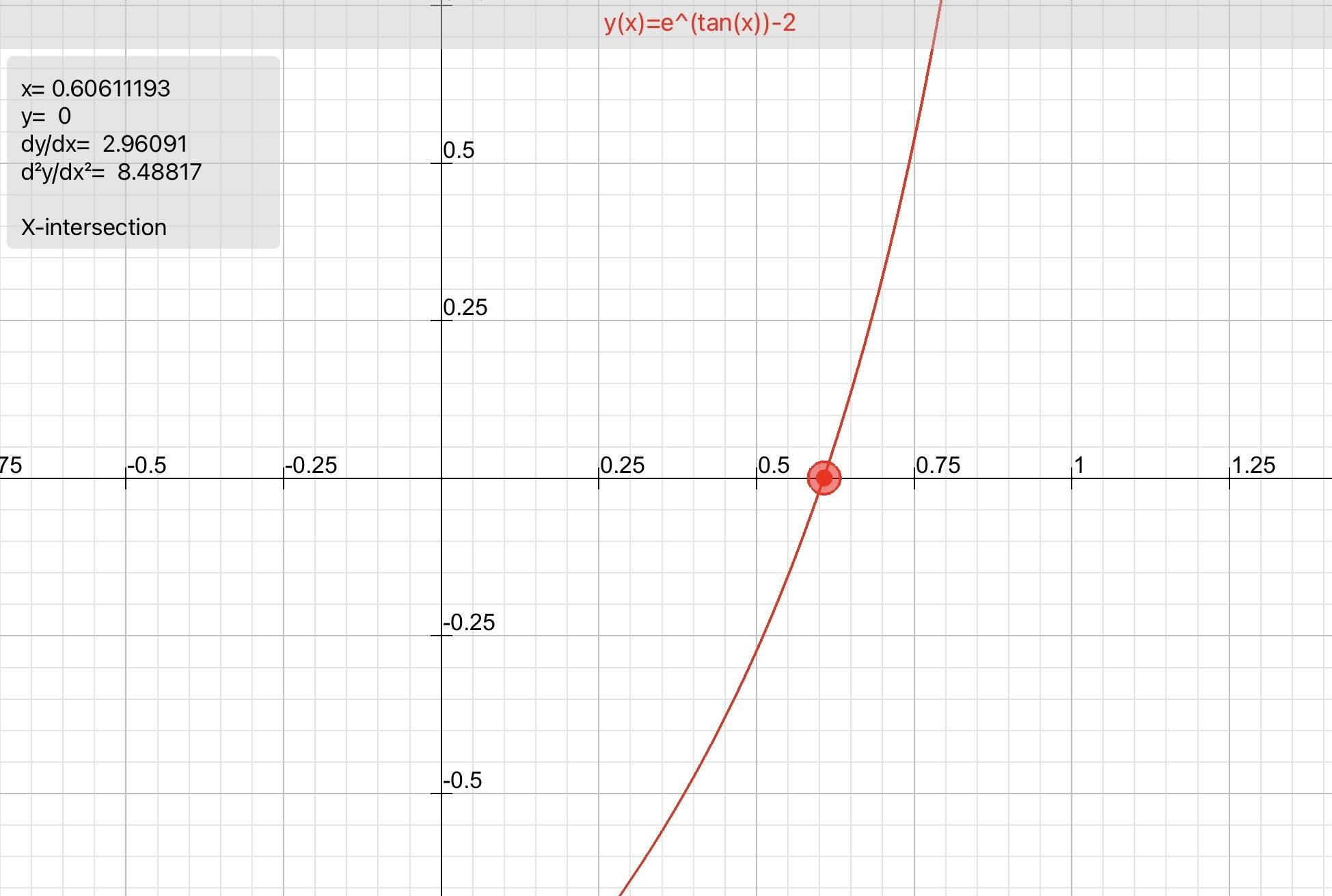# -apc.2.1.06 crosses the x-axis at one point in the interval [0,1]

• MHB
Gold Member
MHB
$\tiny{APC.2.1.06}$
The graph of $y=e^{\tan x}-2$ crosses the x-axis at one point in the interval [0,1]
What is the slope of the graph at this point.
a, 0.606 b. 2 c, 2.242 d.2.961 e.3.747

[d]
$\begin{array}{rll} \textit{given} &e^{\tan \:x}-2 &(1)\\ \textit{rewrite} &e^{\tan x}=2 &(2) \\ \textit{e thru} &\tan x=\ln 2 &(3)\\ \textit{isolate x } &x=\arctan \ln 2+\pi n &(4)\\ \textit{[0,1] } &\arctan(\ln 2)=0.606 &(5)\\ \textit{m at x} &y_m=e^{\tan (x)}\sec ^2(x) &(6)\\ \textit{m} &y_m(0.606)=2.96 &(7) \end{array}$

it took me 2 hours to do this
must be a better way

if its correct:unsure:

skeeter
fyi, this is a calculator active problem …HOI
$\tiny{APC.2.1.06}$
The graph of $y=e^{\tan x}-2$ crosses the x-axis at one point in the interval [0,1]
What is the slope of the graph at this point.
a, 0.606 b. 2 c, 2.242 d.2.961 e.3.747

[d]
$\begin{array}{rll} \textit{given} &e^{\tan \:x}-2 &(1)\\ \textit{rewrite} &e^{\tan x}=2 &(2) \\ \textit{e thru} &\tan x=\ln 2 &(3)\\ \textit{isolate x } &x=\arctan \ln 2+\pi n &(4)\\ \textit{[0,1] } &\arctan(\ln 2)=0.606 &(5)\\ \textit{m at x} &y_m=e^{\tan (x)}\sec ^2(x) &(6)\\ \textit{m} &y_m(0.606)=2.96 &(7) \end{array}$

it took me 2 hours to do this
must be a better way

if its correct:unsure:
You mean "given $e^{\tan(x)}-2= 0$". Otherwise your second line makes no sense!

Gold Member
MHB
ok guess I was assuming that
fyi, this is a calculator active problem …

View attachment 11165
what graphing program is that from?
doesnt look like Desmos

skeeter
ok guess I was assuming that

what graphing program is that from?
doesnt look like Desmos

Good Grapher Pro app on my ipad.